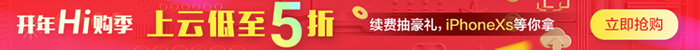#opencv 2 computer vision application programming第二章翻译

1. 云栖社区>
2. 博客>
3. 正文## opencv 2 computer vision application programming第二章翻译

lovedan 2013-04-30 15:17:00 浏览260 评论0

【概述】

【处理像素值】

``` 1 void salt(cv::Mat &image, int n){
2     for(int k=0; k<n; k++){
3         //rand()是MFC随机函数生成器
4         //在Qt中请使用qrand()
5         int i=qrand()%image.cols;
6         int j=qrand()%image.rows;
7
8         if(image.channels()==1){//对应灰度图像
9             image.at<uchar>(j, i)=255;
10         }else if(image.channels()==3){//彩色图像
11             image.at<cv::Vec3b>(j, i)=255;
12             image.at<cv::Vec3b>(j, i)=255;
13             image.at<cv::Vec3b>(j, i)=255;
14         }
15     }
16 }```

```1 //open the image
2 cv::Mat image = cv::imread("boldt.jpg");
3
4 //call function to add noise
5 salt(image, 3000);
6
7 //display image
8 cv::namedWindow("Image");
9 cv::imshow("Image", image);```

``` 1 #include <cv.h>
2 #include <highgui.h>
3
4 using namespace std;
5 using namespace cv;
6
7 void salt(Mat& image, int n){
8     for(int k=0; k<n; k++){
9         int i=rand()%image.cols;
10         int j=rand()%image.rows;
11
12         if(image.channels()==1){
13             image.at<uchar>(j, i)=255;
14         }else if(image.channels()==3){
15             image.at<Vec3b>(j, i)=255;
16             image.at<Vec3b>(j, i)=255;
17             image.at<Vec3b>(j, i)=255;
18         }
19     }
20 }
21
22 int main(){
24     salt(image, 3000);
25     namedWindow("Image");
26     imshow("Image", image);
27     waitKey(0);
28 }```

【更多】

cv::Mat_<uchar>im2=image;//im2 refers to image
im2(50, 100)=0;//access to row 50 and column 100

operator()方法和at方法等效。

【同见】

【用指针扫描图像】

【准备阶段】

【如何做】

void colorReduce(cv::Mat &image, int div=64);

，即：输入图像的像素值被这个函数修改了。更多的输入输出参数参见【更

void colorReduce(cv::Mat &image, int div=64){
int nl=image.rows;//行数
//每一行元素总数
int nc=image.cols*image.channels();

for(int j=0; j<nl; j++){
//获取第j行的地址
uchar* data=image.ptr<uchar>(j);
for(int i=0; i<nc; i++){
//处理每个像素
data[i]=data[i]/div*div+div/2;
//处理像素过程结束
}//每一行处理完毕
}
}

//读入图像
//处理图像
colorReduce(image);
//展示图像
cv::namedWindow("Image");
cv::imshow("Image", image);

``` 1 【更多】
2 使用cv::Mat的at方法有时候很笨重。因为返回的类型必须被规定为模板参
3
4 数。当矩阵类型未知时，使用cv::Mat的子类cv::Mat_类是可以的。这个类
5
6 定义了少量额外的方法但没有定义更多的数据属性，因此一个指向类的指针
7
8 或者引用可以被直接地转化成另外的类。在其他的额外方法中，operator()
9
10 方法允许直接访问矩阵元素。因此，如果图像是指向uchar类型的引用，那
11
12 么可以这样写：
13     cv::Mat_<uchar>im2=image;//im2 refers to image
14     im2(50, 100)=0;//access to row 50 and column 100
15 由于cv::Mat_类的元素类型在变量创建的时候已经声明过了,operator()方
16
17 法在编译的时候知道返回值的类型。不同于写入的时候变shorter，
18
19 operator()方法和at方法等效。
20
21 【同见】
22 编写高效扫描图像的循环方法
23
24 【用指针扫描图像】
25 在大多数图像处理过程中，人们为了执行计算需要处理图像的所有像素。考
26
27 虑到图像中如此多的像素数量，高效的方式很重要。这一部分，以及下一部
28
29 分，会向你展示扫描图像的不同的循环的实现方式。这一部分使用指针来计
30
31 算。
32
33 【准备阶段】
34 我们通过完成一个加单的任务来实现图像扫描过程：减少一副图像中的颜色
35
36 的数量
37
38 彩色图像由3个通道(3-channels)的像素组成.每个像素的每个通道对应着三
39
40 原色中的强度值。由于这些值都是8位的无符号字符类型值，颜色数量的总
41
42 数是256*256*256，大于1.6亿个颜色。所以为了减少分析时的复杂度，有时
43
44 候减少图像的数量是有用的。实现这个目标的一个简答方法是，把RGB空间
45
46 分割成大小相等的立方体。例如，你想在每个维度把颜色数量减少8个，那
47
48 么你得到的颜色总是就是32*32*32.原图中每个颜色也就变成了颜色衰减后
49
50 的图中所属立方体中心位置的颜色值。
51
52 基本的颜色减少算法是简单的。若N是减少因子，那么对于图像中每个像素
53
54 和每个像素中的通道，都要整除N（余数会丢失）。然后把结果乘以N，所得
55
56 到的会比原来输入的图像要小。加上N/2，你会得到两者的中间值。持续着
57
58 一过程，你会得到总数为(256/N)^3的可能的颜色数量。
59
60 【如何做】
61 颜色衰减函数这样声明：
62      void colorReduce(cv::Mat &image, int div=64);
63 用户提供一张图片和每个通道的衰减因子。这里，此过程恰到好处的完成了
64
65 ，即：输入图像的像素值被这个函数修改了。更多的输入输出参数参见【更
66
67 多】部分。
68
69 这个过程很简单，通过创建一个遍历像素的所有值的一个double循环以实现
70
71 ：
72 void colorReduce(cv::Mat &image, int div=64){
73     int nl=image.rows;//行数
74     //每一行元素总数
75     int nc=image.cols*image.channels();
76
77     for(int j=0; j<nl; j++){
78         //获取第j行的地址
79         uchar* data=image.ptr<uchar>(j);
80         for(int i=0; i<nc; i++){
81             //处理每个像素
82             data[i]=data[i]/div*div+div/2;
83             //处理像素过程结束
84         }//每一行处理完毕
85     }
86 }
87 这个函数可以用下面代码测试:
88     //读入图像
90     //处理图像
91     colorReduce(image);
92     //展示图像
93     cv::namedWindow("Image");
94     cv::imshow("Image", image);```

【如何起作用的】

8的倍数的时候能高效处理。明显地，若没有增加这些额外的行那么有效的

，而tep数据属性以字节为单位给定了图像的宽度。即使你的图像不是uchar

（例如，对与3通道的短整型矩阵（CV_16SC3），elemSize会返回6）。图像

3）。最终，total这个方法返回矩阵中像素总数。

int nc=image.cols*image.channels();

uchar* data=image.ptr<uchar>(j);

*data++=*data/div*div+div/2;        //发现书上写错了。
【其他颜色衰减公式】
data[i]=data[i]-data[i]%div+div/2;

当然亦可在函数中增加参数，不在原图上做修改：

``` 1 #include <cv.h>
2 #include <highgui.h>
3
4 using namespace std;
5 using namespace cv;
6
7 void colorReduce(const Mat &image, Mat &result, int div=64){
8     int nl=image.rows;
9     int nc=image.cols*image.channels();
10     result.create(image.rows, image.cols, image.type());
11     for(int j=0; j<nl; j++){
12         const uchar* data_in=image.ptr<uchar>(j);
13         uchar* data_out=result.ptr<uchar>(j);
14         for(int i=0; i<nc; i++){
15             data_out[i]=data_in[i]/div*div+div/2;
16         }
17     }
18 }
19
20 int main(){
22     Mat result;
23     colorReduce(image, result);
24     namedWindow("Image");
25     imshow("Image", result);
26     waitKey(0);
27 }```

``` 1 #include <cv.h>
2 #include <highgui.h>
3
4 using namespace std;
5 using namespace cv;
6
7 void colorReduce(Mat &image, int div=64){
8     int nl=image.rows;
9     int nc=image.cols*image.channels();
10     if(image.isContinuous()){
11         nc=nc*nl;
12         nl=1;
13         //or:image.reshape(1, image.cols*image.rows);
14         //then int nl=image.rows; and int nc=image.cols;
15     }
16     for(int j=0; j<nl; j++){
17         uchar* data=image.ptr<uchar>(j);
18         for(int i=0; i<nc; i++){
19             data[i]=data[i]/div*div+div/2;
20         }
21     }
22 }
23
24 int main(){
26     colorReduce(image);
27     namedWindow("Image");
28     imshow("Image", image);
29     waitKey(0);
30 }```

【低级指针计算】

```1 uchar* data=image.data;
2 data+=image.step; //从当前行跳转到要处理的下一行```

【【用迭代器处理图像衰减】】

【图像扫描至邻居法】

``` 1 #include <cv.h>
2 #include <highgui.h>
3
4 using namespace std;
5 using namespace cv;
6
7 void sharpen(const Mat& image, Mat &result){
8     result.create(image.size(), image.type());
9     imshow("image", image);
10     for(int j=1; j<image.rows-1; j++){
11         const uchar* previous=image.ptr<const uchar>(j-1);
12         const uchar* current=image.ptr<const uchar>(j);
13         const uchar* next=image.ptr<const uchar>(j+1);
14         uchar* output=result.ptr<uchar>(j);
15         for(int i=1; i<image.cols*3; i++){//此处书上写为i<image.cols-1发现只显示1/3宽度
16             *output=saturate_cast<uchar>(5*current[i]-current[i-1]
17                     -current[i+1]-previous[i]-next[i]);
18             output++;
19         }
20     }
21     result.row(0).setTo(Scalar(0));
22     result.row(result.rows-1).setTo(Scalar(0));
23     result.col(0).setTo(Scalar(0));
24     result.col(result.cols-1).setTo(Scalar(0));
25 }
26 int main(){
28     Mat result;
29     sharpen(image, result);
30     namedWindow("Image");
31     imshow("Image", result);
32     waitKey(0);
33     return 0;
34 }```

使用系统函数filter2D：

```#include <cv.h>
#include <highgui.h>

using namespace std;
using namespace cv;

void sharpen2D(const Mat& image, Mat &result){
Mat kernel(3, 3, CV_32F, Scalar(0));
kernel.at<float>(1,1)=5.0;
kernel.at<float>(0,1)=-1.0;
kernel.at<float>(2,1)=-1.0;
kernel.at<float>(1,0)=-1.0;
kernel.at<float>(1,2)=-1.0;
filter2D(image, result, image.depth(), kernel);
}
int main(){
if(!image.data) return -1;
Mat result;
sharpen2D(image, result);
namedWindow("Image");
imshow("image", image);
imshow("result", result);
waitKey(0);
return 0;
}```

``` 1 /*
2  *图像合成
3  *要求大小一样才可以
4  */
5 #include <cv.h>
6 #include <highgui.h>
7
8 using namespace std;
9 using namespace cv;
10
11 int main(){
14     Mat result;
15     addWeighted(image1, 0.7, image2, 0.9, 0., result);
16 //    imshow("image", image);
17     imshow("result", result);
18     imwrite("C:/testdir/we.jpg", result);
19     waitKey(0);
20     return 0;
21 }```

``` 1 /*
2  *图像合成
3  *在指定区域合成
4  */
5 #include <cv.h>
6 #include <highgui.h>
7
8 using namespace std;
9 using namespace cv;
10
11 int main(){
14     Mat imageROI=image(Rect(600, 1300, logo.cols, logo.rows));
15     addWeighted(imageROI, 1.0, logo, 0.3, 0., imageROI);
16     imshow("result", image);
17     imwrite("C:/testdir/me4.jpg", image);
18     waitKey(0);
19     return 0;
20 }```

【云栖快讯】一站式开发者服务，海量学习资源免费学  详情请点击

lovedan

+ 关注